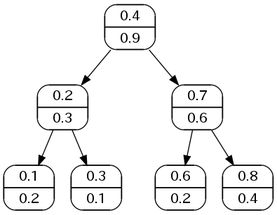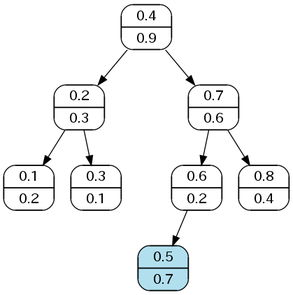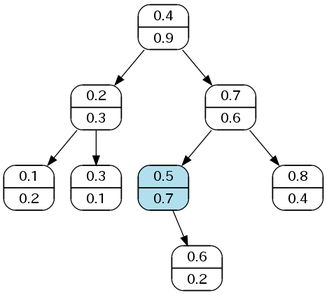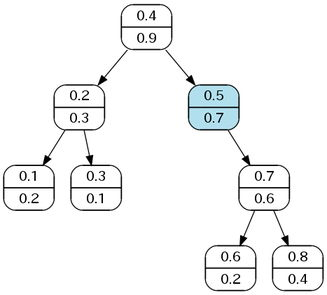시간 제한 메모리 제한 제출 정답 맞은 사람 정답 비율
2.5 초 512 MB 2 1 1 50.000%

## 문제

You insert $N$ random elements with real-valued keys into a treap. Compute the probability that the height of the treap becomes $h$ ($h = 0, 1, \ldots, N-1$).

A treap is a randomized binary search tree. Each node has a key and a priority. In this problem, assume that both values are real values between 0 and 1. In a treap, the following two conditions are always satisfied:

1. The condition about the keys:
• For each node, its key is greater than the keys of its left child and the descendants of its left child.
• For each node, its key is smaller than the leys of its right child and the descendants of its right child.
2. The condition about the priorities:
• For each node, its priority is greater than the priorities of its children.

The following figure shows an example of a treap. In each node, the upper half contains the key, and the lower half contains the priority.When you insert a node with key $x$, do the following:

1. Determine the priority of the new node, $p$, uniformly at random between 0 and 1.
2. First, as with a standard binary search tree, ignore the priorities and insert a node based on its key.
3. Then, while the condition about the priority is not satisfied, perform rotations and move the new node upward.

In the following figures, a node with key 0.5 and priority 0.5 is inserted into the treap shown above.You choose $N$ keys uniformly at random between 0 and 1, and insert them one by one into an empty treap. For each $h = 0, 1, \ldots, N - 1$, print the probability that the final height of the treap becomes $h$. The real numbers are sufficiently precise, and the probability that two random numbers become the same is zero. The height is defined as the maximum number of edges you must pass through to go from a leaf to the root.

## 입력

You are given an integer $N$ ($1 \le N \le 3 \cdot 10^4$).

## 출력

Print $N$ lines. On the $i$-th line, print the probability that the height becomes $i-1$. The absolute error must be at most $10^{-5}$.

## 예제 입력 1

1


## 예제 출력 1

1.0


## 예제 입력 2

2


## 예제 출력 2

0.00000
1.00000